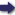(London :  Kegan Paul, Trench, Trübner & Co.,  1910.)

 Tools

## Search this bookPrev Page 141 Next``` CHAPTER XIII. 141 In a lexicographical work to which the author Onthear- ^^ rangement J V (? Haribhatta) has given his own name, the feet Quotation' -^-V from Hari- composed of three Iccghu or guru are called by single i^hatta. consonants, which in the following diagram are written on their left:— Diagram, m < < < sixfold {i.e. containing six mdtrd). y I < < hastin. r < I < kdma. t < < \ {1 lacuna). s I 1 < jvalana. j I < I madhya. hh < \ I parvata. n \ \ \ threefold {i.e. containing three mdtrd). By means of these signs the author teaches how to construct these eight feet by an inductive method (a kind of algebraic permutation), saying : "Place one of the two kinds (guru and laghu) in the first line unmixed (that would be <<<, if we Page68. begin with a guru). Then mix it with the second kind, and place one of this at the beginning of the second line, whilst the two other elements are of the first kind (| < <). P'hen place this element of admix¬ ture in the middle of the third line ( < | < ), and lastly at the end of the fourth line (< <|). Then you have finished the first half, "Further, place the second kind in the lowest line, unmixed (| | |), and mix up with the line above it one of the first kind, placing it at the beginning of the line (< I 1), then in the middle of the next following line (I < I), aud lastly at the end of the next following line (I I <). Then the second half is finished, and all the possible combinations of three mdtra have been ex¬ hausted." < < < I < < \ First half. ^' ' 1 ! i 3- < I < 7- < M I } i 8. I I I i 5. , I < , Second half. 4. < < I ^ \ 8. 1 I I This system of composition or permutation is correct. ```Prev Page 141 Next